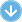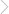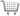128 Pages## Walker Maths 3.5 Complex Numbers

### By Charlotte Walker, Victoria Walker | Copyright Year:2020 | ISBN-13: 9780170447058

Published:30/01/2020Walker Maths is a series of single standard workbooks containing high-quality, up to date material at NCEA Mathematics levels 1, 2 and 3.

The well-designed, write-on workbooks contain teaching material, including relevant formulae, and ample practice exercises along with sample tasks and questions. The workbooks reflect the content and style of the new standards, and allow teachers total flexibility in course design for students at all levels.

As a single standard series, Walker Maths offers Maths department the ability to buy titles all at once, or throughout the year as required.

A Walker Maths Digital Teacher Resource is available for \$9.95 per year for a single download. Each Digital Teacher Resource includes a Walker Maths eBook/projection file. Plus a selection of
• Worksheets
• Extra questions
• Teacher notes
• Videos
• Puzzle sheets
• Practice quizzes
• Worked solutions

### CONTENTS

Glossary

Revision and useful background
1 Polynomial equations
Expanding three brackets
Roots of equations and use of the discriminant
2 Circles
3 Trigonometry
Trigonometric functions
Exact values for trigonometric functions: special triangles
Another way of viewing trigonometric functions
Putting it together

Groups of numbers

Surds
Rules for surds
Simplifying expressions containing surds
Rationalising and more simplifying of expressions containing
surds
Equations involving surds

Polynomials
Completing the square
Long division
Factor and remainder theorems
1 The factor theorem
2 The remainder theorem

Complex numbers
Imaginary numbers
Manipulation of imaginary numbers
Complex numbers — the basics
Complex numbers and Argand diagrams
Manipulation of complex numbers
Multiplication
Division
Mixing it up
The modulus
Polynomials again
The fundamental theorem of algebra
Cubics
Polar coordinates
Conversion from Cartesian form to polar form
Conversion from polar form to Cartesian form
Conversion using a calculator
Multiplying and dividing complex numbers in polar form
Mixing it up
Powers of complex numbers in polar form: de Moivre’s theorem
Solving polynomial equations using de Moivre’s theorem
Finding the locus of complex numbers subject to restrictions
1 Where the real and/or imaginary part of the complex number is restricted
2 Where the modulus of the complex number is restricted
3 Where the argument of the complex number is restricted